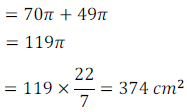CBSE Board NCERT Solutions for Class 10th Mathematics Chapter 13 Surface Areas and Volumes
CBSE NCERT Solutions for Class Ten Mathematics Chapter 13 Surface Areas and Volumes

CBSE  NCERT Solutins For Class 10 Mathematics. Exercise 13.1, Exercise 13.2, Exercise 13.3, Exercise 13.4, Exercise 13.5

NCERT Solutions for Class 10th Maths Chapter 13 Surface Areas And Volumes – Mathematics
Exercise 13.1 (NCERT)

Question 1: 2 cubes each of volume 64 cm3 are joined end to end. Find the surface area of the resulting cuboid.
Solution: Side of cube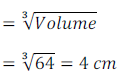Length of new cuboid = 8 cm, height = 4 cm, width = 4 cm

Surface Area can be calculated as follows: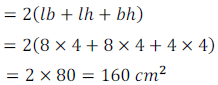Alternate Method:

Surface area of cube = 6 x side2

When two cubes are joined end to end, then out of 12 surfaces; two surfaces are lost due to joint. Thus, we need to take surface area of 10 surfaces and hence surface area can be given as follows:

= 10 x side 2

= 10 x 42 = 160 cm2

Question 2: A vessel is in the form of a hollow hemisphere mounted by a hollow cylinder. The diameter of the hemisphere is 14 cm and the total height of the vessel is 13 cm. Find the inner surface area of the vessel.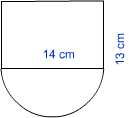Height of cylindrical portion = 13 – 7 = 6 cm

Curved surface are of cylindrical portion can be calculated as follows: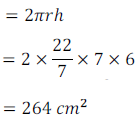Curved surface area of hemispherical portion can be calculated as follows: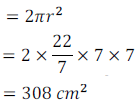Total surface are = 308 + 264 = 572 sq cm

Question 3: A toy is in the form of a cone of radius 3.5 cm mounted on a hemisphere of same radius. The total height of the toy is 15.5 cm. Find the total surface area of the toy.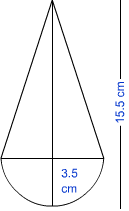Solution: Radius of cone = 3.5 cm, height of cone = 15.5 – 3.5 = 12 cm

Slant height of cone can be calculated as follows: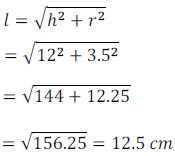Curved surface area of cone can be calculated as follows: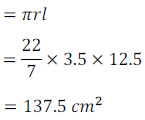Curved surface area of hemispherical portion can be calculated as follows:Hence, total surface area = 137.5 + 77 = 214.5 sq cm

Question 4: A cubical block of side 7 cm is surmounted by a hemisphere. What is the greatest diameter the hemisphere can have? Find the surface area of the solid.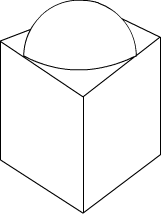Solution: The greatest diameter = side of the cube = 7 cm

Surface Area of Solid = Surface Area of Cube – Surface Area of Base of Hemisphere + Curved Surface Area of hemisphere

Surface Area of Cube = 6 x Side2

= 6 x 7 x 7 = 294 sq cm

Surface Area of Base of Hemisphere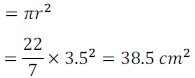Curved Surface Area of Hemisphere = 2 x 38.5 = 77 sq cm

Total Surface Area = 294 – 38.5 + 77 = 332.5 sq cm

Question 5: A hemispherical depression is cut out from one face of a cubical wooden block such that the diameter d of the hemisphere is equal to the edge of the cube. Determine the surface area of the remaining solid.
Solution: This Question can be solved like previous Question . Here the curved surface of the hemisphere is a depression, unlike a projection in the previous Question
Total Surface Area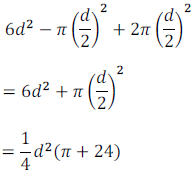Question 6: A medicine capsule is in the shape of a cylinder with two hemispheres stuck to each of its ends. The length of the entire capsule is 14 mm and the diameter of the capsule is 5 mm. Find its surface area.
Solution:

Height of Cylinder = 14 – 5 = 9 cm, radius = 2.5 cm

Curved Surface Area of Cylinder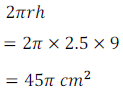Curved Surface Area of two HemispheresTotal Surface Area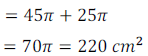Question 7: A tent is in the shape of a cylinder surmounted by a conical top. If the height and diameter of the cylindrical part are 2.1 m and 4 m respectively, and the slant height of the top is 2.8 m, find the area of the canvas used for making the tent. Also, find the cost of the canvas of the tent at the rate of Rs 500 per m2. (Note that the base of the tent will not be covered with canvas.)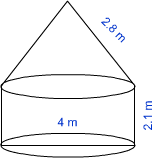Solution: Radius of cylinder = 2 m, height = 2.1 m and slant height of conical top = 2.8 m

Curved Surface Area of cylindrical portionCurved Surface Area of conical portionTotal CSA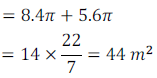Cost of canvas = Rate x Surface Area

= 500 x 44 = Rs. 22000

Question 8: From a solid cylinder whose height is 2.4 cm and diameter 1.4 cm, a conical cavity of the same height and same diameter is hollowed out. Find the total surface area of the remaining solid to the nearest cm2.
Solution: Radius = 0.7 cm and height = 2.4 cm

Total Surface Area of Structure = Curved Surface Area of Cylinder + Area of top of cylinder + Curved Surface Area of Cone

Curved Surface Area of Cylinder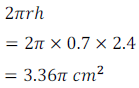Area of topSlant height of cone can be calculated as follows: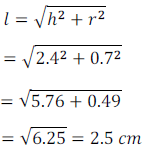Curved Surface Area of Cone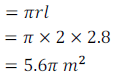Hence, remaining surface area of structure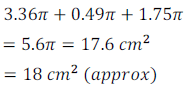Question 9: A wooden article was made by scooping out a hemisphere from each end of a solid cylinder, as shown in figure. If the height of the cylinder is 10 cm, and its base is of radius 3.5 cm, find the total surface area of the article.
Solution:

Radius = 3.5 cm, height = 10 cm

Total Surface Area of Structure = CSA of Cylinder + CSA of two hemispheres

Curved Surface Area of Cylinder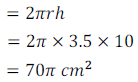Surface Area of Sphere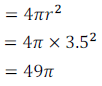Total Surface Area